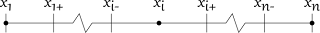## Saturday, 22 December 2012

### Discretising Base Traffic Model Equations

This post is a followup of these earlier posts. Here we discretise the traffic model equations to let us solve them numerically. Here are the model equations (including boundary conditions) all together in one place:
$$q = \rho v,$$ $$\frac{\partial \rho}{\partial t} + \frac{\partial q}{\partial x} = S(x,t),$$ $$v=v_f ( 1 - \rho/\rho_j ),$$ $$S(x,t) = 0,$$ $$\rho(x,0) = 0,$$ and $$\rho(0,t) = \rho_j/4.$$ where
$$q$$ = traffic flow rate (veh/s) past the point;
$$\rho$$ = vehicular density (veh/m);
$$v$$ = vehicle speed (m/s);
$$\partial$$ indicates partial differentiation;
$$t$$ = time (s);
$$x$$ = distance (m) along road;
$$S$$ = vehicles entering (+ve) or leaving (-ve) the road (veh/m/hr);
$$v_f$$ = free flow speed (m/s); and
$$\rho_j$$ = jam density (veh/m), the average vehicles per metre in stationary traffic.

Note that we've set $$S(x,t)$$ to zero. This means that no vehicles are leaving or entering the road apart from at the ends.

The presence of the very simple equation for flow, $$q$$, means that we can eliminate flow from the equations altogether. When a value for flow is needed, we can just multiply density and speed together. As we progress, we may (or may not) find it desirable to do the same with speed. With flow removed, the main equation becomes: $$\frac{\partial \rho}{\partial t} + \frac{\partial \rho v}{\partial x} = S(x,t).$$ My favourite discretisation method is to use control volumes - the finite volume method. The first step is to divide the domain into cells, or control volumes. Here is how we will do that:Discretisation grid for our traffic equations, showing coordinates for cells 1, i and n.
where a trailing minus sign indicates the left boundary, and a trailing plus sign indicates the right boundary. Because the right boundary of one cell is the left boundary of the next cell, we have $$x_{i+} = x_{i+1-}$$. Dots indicate the location used to calculate representative model values contents for each cell.

There is also a time dimension, and we'll refer to the current time with $$t_c$$, the target, or future, time with $$t_f$$. The time step of our discretisation is given by $$t_f - t_c = \Delta t$$.

We will discretise our density equation by integrating it over the volume of each cell, 1 to $$n$$. Since we have two dimension, $$x$$ and $$t$$, the integration over the volume involves two integrals.

## Body Cells: 2 to n-1

First we'll integrate over space: $$\int_{x_{i-}}^{x_{i+}}\frac{\partial \rho}{\partial t}\,dx + \int_{x_{i-}}^{x_{i+}}\frac{\partial \rho v}{\partial x}\,dx = 0.$$ The first integral is approximated using the rectangle rule with one rectangle using the midpoint approximation ($$x=x_i$$). The second integral simplifies thanks to the fundamental theorem of calculus. Applying those (and explicitly writing the function arguments for $$\rho$$ and $$v$$), we get: $$(x_{i+}-x_{i-}) \frac{\partial \rho(x_i,t)}{\partial t} + \left[\rho(x,t) v(x,t)\right]_{x_{i-}}^{x_{i+}} = 0.$$ Now we'll apply the time integral from the current time, $$t_c$$, to the future time, $$t_f$$. For the second integral, we use the rectangle rule, with top left corner approximation ($$t=t_c$$): $$(x_{i+}-x_{i-}) \left[\rho(x_i,t)\right]_{t_c}^{t_f} + (t_f-t_c) \left[\rho(x,t_c) v(x,t_c)\right]_{x_{i-}}^{x_{i+}} = 0.$$ Next, we rearrange it to have the density at the future time on the left, and everything else on the right: $$\rho(x_i,t_f) = \rho(x_i,t_c) - \frac{t_f-t_c}{x_{i+}-x_{i-}}\left(\rho(x_{i+},t_c) v(x_{i+},t_c) - \rho(x_{i-},t_c) v(x_{i-},t_c)\right).$$

## Left Boundary Cell: 1

The left boundary equation doesn't need to be obtained by integrating as it is given by the existing boundary condition: $$\rho(0,t) = \rho_j/4.$$

## Right Boundary Cell: n

For the right boundary cell, we can reuse the equation from the body cells, but updated to refer to the last cell's coordinates ($$i\rightarrow n, x_{i+}\rightarrow x_{n}$$): $$\rho(x_n,t_f) = \rho(x_n,t_c) - \frac{t_f-t_c}{x_n-x_{n-}}\left(\rho(x_n,t_c) v(x_n,t_c) - \rho(x_{n-},t_c) v(x_{n-},t_c)\right).$$

The next step in this thread will be solving these discretised equations.